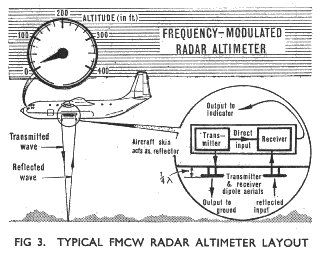Importance

FMCW radars main applications are as altimeters, placed in aircraft, but also in the investigation of atmosphere, thanks to the sensitivity and spatial resolution that they provide in comparison with their pulsed counterparts. In addition, the FMCW radars are more compact and less expensive than the pulsed ones, which make them more suitable for aerial applications.In this post we are going to analyze the backscattering effect, a very important phenomenon considered in many applications, as we will describe later.

In a previous post, we saw a Matlab tutorial to study the the main theoretical principles of the FMCW Radar, summarized in the image below:As shown in the previous image, the echo from a single target will arrive with a time delay of$\Delta{t}=\frac{2R}{c}$

where c is the speed of light and R is the range or distance to the target.

Assuming a stationary target, the beat frequency is:$f_b=\frac{2R}{c}\cdot{PRF}\cdot{\Delta{f}}$

If the target is moving, we would superimpose the Doppler frequency shift, fd, on the beat frequency.

Signal to Noise Ratio and Clutter Backscatter Coefficient

The general expression for a radar SNR is:$SNR=\frac{P_t\cdot{G^2}\cdot{\lambda^2}\cdot{\sigma}}{(4\pi)^3\cdot{R^4}\cdot{kT_0 B_0}\cdot{F_n}\cdot{L_s}}$

where${\sigma}$ is the radar cross section (RCS) , k is the Boltzmann’s constant, T0=290K is the standard temperature, and$B_n$ is the noise bandwidth.

The processing gain due to the beat frequency for FMCW radars is$\frac{B_n}{PRF}$. This is the pulse compression ratio in a pulsed radar system. Inserting this gain in the previous formula, we can write the SNR as:$SNR=\frac{P_t\cdot{G^2}\cdot{\lambda^2}\cdot{\sigma}}{(4\pi)^3\cdot{R^4}\cdot{kT_0 PRF}\cdot{F_n}\cdot{L_s}}$

The clutter-to-noise ratio (CNR) can be calculated by replacing the RCS  in the SNR equation by  the clutter backscatter coefficient of the illuminated surface,${\sigma_{clutter}}$.

If we know the beat frequency and the range resolution, we can use a resolution cell for the illuminated surface, instead of a swath. Approximating this cell by a rectangle, we have:${\sigma_{clutter}=\Delta{r_{r,ground}}\cdot{\theta_{a}}\cdot{R_{far}}\cdot{\sigma_0}}$

where${\sigma_0}$ is the RCS per unit area of the illuminated cell. Combining this equation with the SNR equation, we cam determine the CNR as:$CNR=\frac{P_t\cdot{G^2}\cdot{\lambda^2}\cdot{\Delta{r_{r,ground}}}\cdot{\theta_{a}}\cdot{\sigma_0}}{(4\pi)^3\cdot{R^3}\cdot{kT_0 PRF}\cdot{F_n}\cdot{L_s}}$

Bear in mine, the importance of defining what a clutter and what a target is. The targets usually refer to point scatterers and the clutters to extended scatterers (a surface/volume). The analysis of the backscatter from a clutter is made with the aim of separating it from the information signal.

Sources

This post was requested by one of our readers, so we hope it was useful and please, don’t hesitate to contact us to suggest more topics or ask questions 🙂